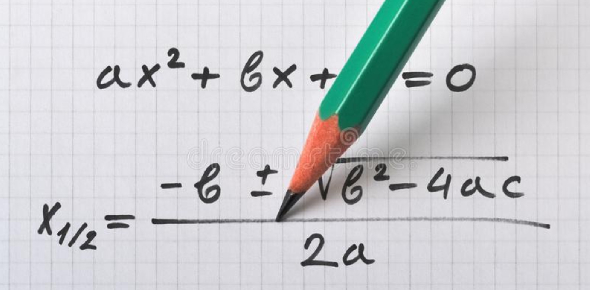10 Questions | Total Attempts: 66Settings.

• 1.
In these questions, two equations are given. You have to solve both the equations and mark the appropriate answer. X^2 = 49 Y^2 − 4y − 21 = 0
• A.

x < y

• B.

X > y

• C.

X ≤ y

• D.

X ≥ y

• E.

X = y or the relationship between x and y cannot be determined

• 2.
In these questions, two equations are given. You have to solve both the equations and mark the appropriate answer. 3x^2 − 13x + 12 = 0 2y^2 − 15y + 28 = 0
• A.

x < y

• B.

X > y

• C.

X ≤ y

• D.

X ≥ y

• E.

X = y or the relationship between x and y cannot be determined

• 3.
In these questions, two equations are given. You have to solve both the equations and mark the appropriate answer.X^2 = 162y^2 − 17y + 36 = 0
• A.

x < y

• B.

X > y

• C.

X ≤ y

• D.

X ≥ y

• E.

X = y or the relationship between x and y cannot be determined

• 4.
In these questions, two equations are given. You have to solve both the equations and mark the appropriate answer.6x^2 + 19x + 15 = 03y^2 + 11y + 10 = 0
• A.

x < y

• B.

X > y

• C.

X ≤ y

• D.

X ≥ y

• E.

X = y or the relationship between x and y cannot be determined

• 5.
In these questions, two equations are given. You have to solve both the equations and mark the appropriate answer.2x^2 − 11x + 15 = 02y^2 − 11y + 14 = 0
• A.

x < y

• B.

X > y

• C.

X ≤ y

• D.

X ≥ y

• E.

X = y or the relationship between x and y cannot be determined

• 6.
In these questions, two equations are given. You have to solve both the equations and mark the appropriate answer.X^2– 5x– 24 = 0   Y^2– 7y– 18 = 0
• A.

x < y

• B.

X > y

• C.

X ≤ y

• D.

X ≥ y

• E.

X = y or the relationship between x and y cannot be determined

• 7.
In these questions, two equations are given. You have to solve both the equations and mark the appropriate answer.8x^2 + 26x + 15 = 0 4y^2 + 24y + 35 = 0
• A.

x < y

• B.

X > y

• C.

X ≤ y

• D.

X ≥ y

• E.

X = y or the relationship between x and y cannot be determined

• 8.
In these questions, two equations are given. You have to solve both the equations and mark the appropriate answer.9x^2 -27x+20=06y^2– 5y + 1 = 0
• A.

x < y

• B.

X > y

• C.

X ≤ y

• D.

X ≥ y

• E.

X = y or the relationship between x and y cannot be determined

• 9.
In these questions, two equations are given. You have to solve both the equations and mark the appropriate answer. 2x^2 + 19x + 45 = 0 2y^2 + 11y + 12 = 0
• A.

x < y

• B.

X > y

• C.

X ≤ y

• D.

X ≥ y

• E.

X = y or the relationship between x and y cannot be determined

• 10.
In these questions, two equations are given. You have to solve both the equations and mark the appropriate answer.X^2– 6x + 9 = 0Y^2– 11y + 24 = 0
• A.

x < y

• B.

X > y

• C.

X ≤ y

• D.

X ≥ y

• E.

X = y or the relationship between x and y cannot be determined

Related TopicsBack to top